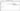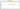Posted on

# Count Cells with NumbersIf you need to count all cells with numbers you can use the Excel COUNT function which counts cells that contain numbers. Its syntax is:
=COUNT(value1, value2,…valueX).
value1, value2,…, valueX  can be cell references, or values typed into the COUNT formula.

Excel COUNT function example – count numbers in cells A2:A7.

1. In the cell where you want to count number enter the following formula =COUNT (A2:A7)
2. Press Enter to complete the formulaThe result will be 3, the number of cells that contain numbers.Cells A2, A4, and A6 aren’t counted, because they contains text

Note: Dates are stored as number, so the COUNT function will include any cells that contain dates.